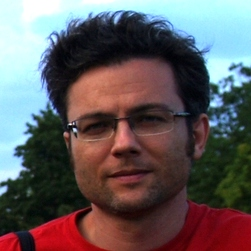# IT.P1.TURINGMACHINE.0.ExComputational Complexity Theory

We are going to compare the computational complexity of algorithms to each other. The computational problems can be grouped together into complexity classes (e.g., NL, P, NP, PSPACE) and some of the those problems are "complete"...
False
registered False
in_cart False
is_course_full False

invitation_only False
can_enroll True

We are going to compare the computational complexity of algorithms to each other. Which one is quicker? Which one uses less memory? How to express time and space complexity in terms of all possible inputs? For this, we need to do theoretical investigations on time and space complexity.

First, let us choose a theoretical model of algorithms: the Turing machine. Then, by using this model, we are going to construct all the necessary concepts that can express the different computations of a Turing machine, the result of such computations, and their time and space complexity. We will get acquinted with several variants of the Turing machine. We will investigate what the relation among those variants is. Can such a variant simulate another one? Is the first one quicker than the latter one?

Here is the main question: Given a computational problem, what is the time and space complexity of the Turing machine that can decide that problem? The computational problems can be grouped together into complexity classes: L, NL, P, NP, PSPACE, EXPTIME, NEXPTIME, EXPSPACE, etc. Furthermore, some of those problems are "complete" for those classes: they can be, for instance, NP-complete, PSPACE-complete, etc. So, computational problems can be characterized: Which one can be decided by using little memory? Which one can be decided fast? Which one is intractable to decide?

Last, by using the above-mentioned tools, we will discuss about the main and not yet solved question of complexity theory: P=NP?

## PREREQUISITES

Skills: algorithmic thinking, logical thinking, abstraction

Recommended knowledge: To known the most important algorithms (search, sorting, and graph algorithms, primality tests). Formal languages, automata.

## Course Staff### Dr. Gergely Kovásznai

Associate professor, Department of Computational Science, Eszterházy Károly University### Ede Troll

Assistant professor, Department of Computational Science, szterházy Károly University### Melinda Pap

Software developer, IoT Research Institute, Eszterházy Károly University

1. Course Number

IT.P1.TURINGMACHINE.0.Ex
2. Classes Start

Feb 01, 2017
3. Estimated Effort

25:00
Enroll